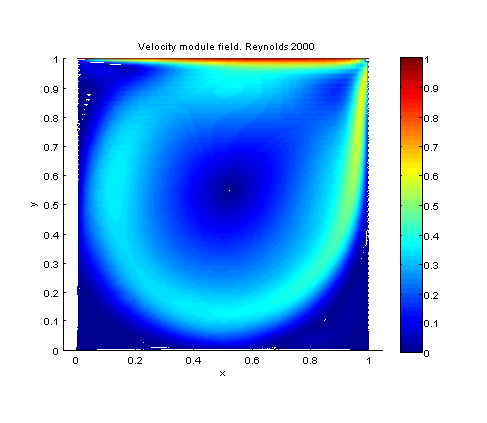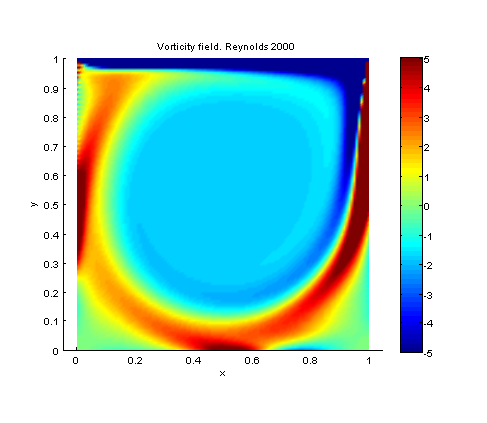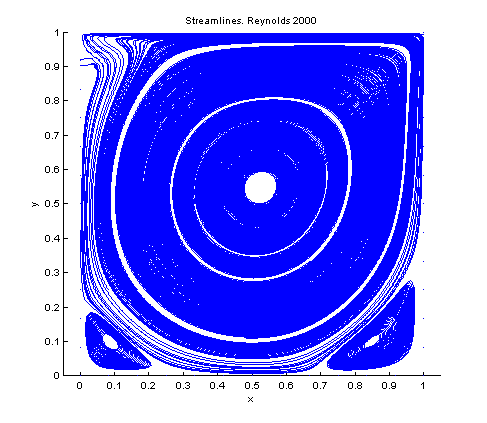# Simulation of Steady Flow of a lid driven cavity

Coded by Paul Valcke and Luis Bernardos. Here we simulate the flow of a rectangular lid driven cavity. We solve the laminar steady Navier Stokes equations, by means of a Newton method using the Jacobian of the equation. Hence, the solved equation (appart from \partial_iu_i=0 of course) is:

\displaystyle u_j\partial_ju_i = -\partial_ip + \frac{1}{Re}\partial_{jj}^2u_i

clear all; figure('OuterPosition',[0, 0, 600, 600]); format compact


# Parameters

Mostly self-explained parameters. This is a Steady calculation, so we implemented a simple strategy of increasing Reynolds, so that we can simulate relatively high Reynolds number re-using a lower reynold number as solution initialization.

%%%% parameters
Re=100; % Starting reynolds number
ReMax = 2000; % Stablished reynolds number
ReNum = 19; % amount of Reynolds steps
Lx = 1; % X Length of domain
Ly = 1; % Y Length of domain
Nx=120; % number of grid nodes in x
Ny=120; % number of grid nodes in y
pts=3; % number of points in finite difference stencils
alpha=1; % Under-relaxation. May help convergence.
maxIter = 200;
resSTOP = 1e-5;

ReStep = (ReMax-Re)/ReNum;

% differentiation
[d.x,d.xx,d.wx,x]=dif1D('fd',0,Lx,Nx,pts);
[d.y,d.yy,d.wy,y]=dif1D('fd',0,Ly,Ny,pts);
[D,l,X,Y,Z,I,NN]=dif2D(d,x,y);
D.lap=D.yy+D.xx;


# Boundary conditions

The boundary conditions of this flow are all-Dirichlet. We impose no-slip conditions everywhere, and we take into consideration that the top wall is moving at a fixed speed of 1.

%%%% preparing boundary conditions
u=(1:NN)'; v=u+NN; p=v+NN;
II=speye(3*NN);

% Condition at the top
dir=[l.ctl;l.ctr;l.left;l.top;l.bot;l.right;l.cbl;l.cbr]; % where to put Dirichlet on u and v
dir2 = [l.top;l.ctl;l.ctr]; % indices of the top wall
loc=[u(dir); v(dir)];

C=II([u(dir);v(dir)],:); % ...     % Dirichlet on u,v


We chose an initial guess that statisfies the boundary conditions, this is good for Newton, and it makes it also very easy to impose the nonhomogeneous boundary conditions in the lop.

% initial guess
U = zeros(NN,1);
U(dir2) = 1; % Here we impose the velocity of the top wall
V=zeros(NN,1);
P=ones(NN,1);
q0=[U(:);V(:);P(:)];

% Newton iterations
disp('Newton loop')
q=q0;
qM=q;
quit=0;count=0;
while ~quit

% the present solution and its derivatives
U=alpha.*q(u) + (1-alpha).*qM(u);
V=alpha.*q(v) + (1-alpha).*qM(v);
P=alpha.*q(p) + (1-alpha).*qM(p);
Ux=D.x*U; Uy=D.y*U;
Vx=D.x*V; Vy=D.y*V;
Px=D.x*P; Py=D.y*P;


This is now the heart of the code: the expression of the nonlinear fonction that should become zero, and just after, the expression of its Jacobian, then the boundary conditions.

    % nonlinear function
f=[-(U.*Ux+V.*Uy)+(D.lap*U)/Re-Px; ...
-(U.*Vx+V.*Vy)+(D.lap*V)/Re-Py; ...
D.x*U+D.y*V];

% Jacobian
A=[-( spd(Ux) + spd(U)*D.x + spd(V)*D.y )+(D.lap)/Re, -spd(Uy), -D.x; ...
-spd(Vx),  -(spd(Vy) + spd(V)*D.y + spd(U)*D.x )+(D.lap)/Re, -D.y; ...
D.x, D.y, Z];

% Boundary conditions
f(loc)=C*(q-q0);
A(loc,:)=C;

Plotting

    % plotting
Module = sqrt((U.^2+V.^2));
surf(X,Y,reshape(Module,Ny,Nx)); view(2); axis equal; shading interp; grid off; colorbar;
xlabel('x'); ylabel('y'); title(sprintf('Velocity module field. Reynolds %0.0f',Re));
drawnow

% convergence test
res=norm(f);
disp([num2str(count) '  ' num2str(res)]);
if (count>maxIter) || (res>1e5); disp('no convergence');break; end
if res<resSTOP && Re < ReMax
fprintf('converged for intermediate Re=%0.0f\n',Re);
if Re < ReMax, Re = Re + ReStep; end
elseif res<resSTOP && Re >= ReMax
quit=1;
fprintf('converged for Final Re=%0.0f\n',Re);
continue;
end

% Newton step
qM = q;
q=qM-A\f;
count=count+1;
end

% Saves the image of the velocity field
set(gcf,'paperpositionmode','auto')
print('-dpng','-r80','CavityLidDrive_Vel.png')

% Plots and saves the image of the vorticity field
Vorticity = -D.y*U + D.x*V;
surf(X,Y,reshape(Vorticity,Ny,Nx)); view(2); axis equal; shading interp; grid off; colorbar;
xlabel('x'); ylabel('y'); title(sprintf('Vorticity field. Reynolds %0.0f',Re));
caxis([-5 5]);
drawnow
set(gcf,'paperpositionmode','auto')
print('-dpng','-r80','CavityLidDrive_Vor.png')

% Plots and saves the image of the streamlines
[startx,starty] = meshgrid(0:Lx/12:Lx,0:Ly/12:Ly);
clf; streamline(X,Y,reshape(U,Ny,Nx),reshape(V,Ny,Nx),startx, starty);axis equal
xlabel('x'); ylabel('y'); title(sprintf('Streamlines. Reynolds %0.0f',Re));
drawnow
set(gcf,'paperpositionmode','auto')
print('-dpng','-r80','CavityLidDrive_SL.png')


# Results

Herein we present three kind of plots: the velocity module, the vorticity and the streamlines. These 3 pictures gives a good insight of the flow. We can observe that a big vortex of the same diameter of the cavity is present. Furthermore, two little counter-rotating vortices appears in the lower corners. This agrees the expected results.Velocity fieldVorticity fieldStreamlines